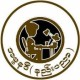# Numbers

Knowing how to read and understand numbers are an essential part of improving your English language skills.

Look in the resources section on learning basic numbers 0-99.

Large numbers:

• 100= one hundred
• 1,000= one thousand
• 10,000= ten thousand
• 100,000= one-hundred thousand
• 1,000,000= one million

• 346= three hundred forty-six
• 739= seven hundred thirty-nine
• 4,692= four thousand six-hundred ninety-two
• 8,115= eight thousand one hundred and fifteen
• 34,999= thirty-four thousand nine-hundred ninety-nine
• 58,301= fifty eight thousand, three-hundred one
• 245,279= two hundred forty five thousand two hundred seventy nine

When talking about numbers or the amount of something you will say… ‘How many

________?’

For example:

1. How many eggs are in a dozen?
2. How many students are in the class?
3. How many people went to the party?
4. When talking about money you will use ‘How much…?”

For example:

1. How much does a box of cookies cost?
2. How much do I owe you?
3. How much does it cost?
4. How much do you make an hour?
5. How much did he pay you?
6. How much do you pay for car insurance?